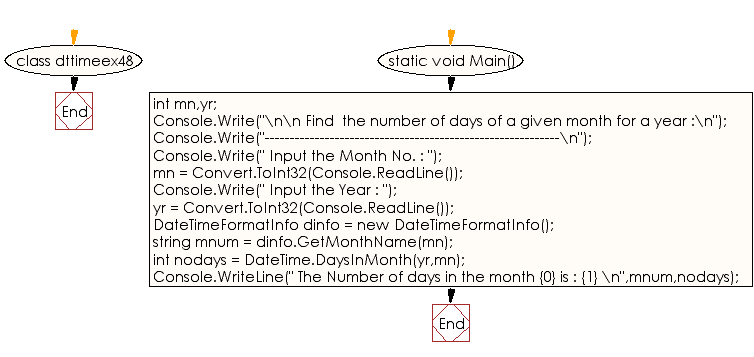﻿ C# - Get the number of days of a given month for a year# C# Sharp Exercises: Get the number of days of a given month for a year

## C# Sharp DateTime: Exercise-48 with Solution

Write a program in C# Sharp to get the number of days of a given month for a year.

Sample Solution:-

C# Sharp Code:

``````using System;
using System.Globalization;

class dttimeex48
{
static void Main()
{

int mn,yr;

Console.Write("\n\n Find  the number of days of a given month for a year :\n");
Console.Write("-----------------------------------------------------------\n");
Console.Write(" Input the Month No. : ");
Console.Write(" Input the Year : ");
DateTimeFormatInfo dinfo = new DateTimeFormatInfo();
string mnum =  dinfo.GetMonthName(mn);
int nodays = DateTime.DaysInMonth(yr,mn);
Console.WriteLine(" The Number of days in the month {0} is : {1} \n",mnum,nodays);

}
}
```
```

Sample Output:

```Find  the number of days of a given month for a year :
-----------------------------------------------------------
Input the Month No. : 6
Input the Year : 2017
The Number of days in the month June is : 30
```

Flowchart:C# Sharp Code Editor:

Improve this sample solution and post your code through Disqus

What is the difficulty level of this exercise?

Test your Programming skills with w3resource's quiz.

﻿